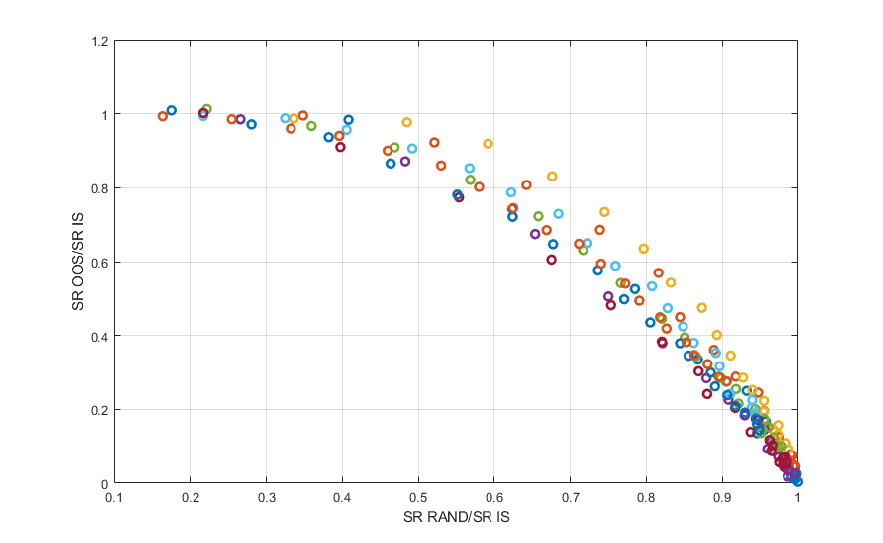This thread is about the question: how to test the robustness of trading strategies. The very nature of a community like this is to introduce an idea for a strategy, which is then iteratively improved by the various contributors of the relevant thread. The iterative process used to improve strategies in of itself increases the risk of overfitting, and poor out-of-sample performance, and by extension a poor real life performance. There are of course many ways to test the robustness of a trading strategy, each with their own advantages, and disadvantages. To start the discussion, I would like to examine the method, where we optimize the trading strategy on random unstructured data, and compare the performance of the strategy on the random data with the real data. To have confidence in the trading strategy we hope to find, that the performance of the trading strategy on real data significantly outperforms the performance of the strategy on random data. To illustrate this point I've generated some simulationed strategies, where I plotted the ratio of the Sharpe Ratio for the strategy applied on random data and the in-sample Sharpe ratio of the simulated strategy, and plotted this ratio against the ratio of the out-of-sample Sharpe ratio and the in-sample Sharpe ratio of the simulated strategy:The conclusion that we can draw from this graph is fairly straightforward: the closer the Sharpe ratio of the strategy applied to random data approaches the Sharpe ratio of the actual trading strategy in-sample, the worse the out-of-sample performance of the strategy will be. For example if a complex strategy with many parameters has a Sharpe ratio of 3, and  the same strategy when optimized on random data results in a Sharpe ratio of 2.8, the resulting ratio of 0.93 would result in an estimated out-of-sample Sharpe ratio of 0.6. This outcome is the main reason why, when only a limited amount of data is available, complex strategies with many parameters are not an option, as the performance on random data is likely to be stellar, and thus unless the Sharpe ratio approaches double digits, the real life performance is all but guaranteed to be a disappointment,